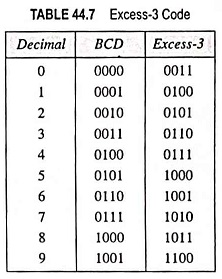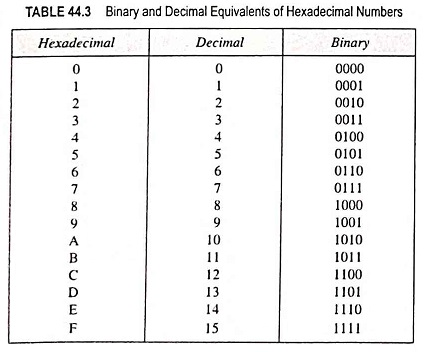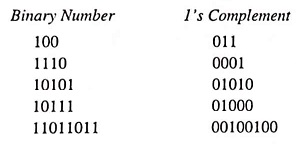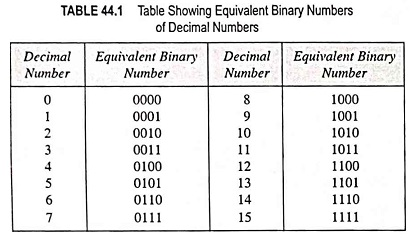# Number System and Codes

## What is Alphanumeric Codes? – ASCII code, EBCDIC Code

What is Alphanumeric Codes? – ASCII code, EBCDIC Code: In order to communicate, we need not only numbers but also letters of alphabet, punctuation marks and other special characters. These codes are called alphanumeric codes. Most of these codes, however, also represent symbols and various instructions necessary for conveying intelligible information. Different codes are used …

## What is Excess-3 Code? (Definition and Examples)What is Excess-3 Code? (Definition and Examples) The excess-3 code, abbreviated as XS-3, is an important 4-bit code sometimes used with binary-coded decimal (BCD) numbers. It possesses advantages in certain arithmetic operations. The excess-3 code for a decimal number can be obtained in the same manner as BCD except that 3 is added to each …

## Hexadecimal Number System (Definition, Conversions & Examples)Hexadecimal Number System (Definition, Conversions & Examples): The binary number system forms the natural choice for the two-state systems. But in this system, the numbers tend to get short rather long. Hence to reduce the length of a given number it is quite common to use hexadecimal system. The hexadecimal number system has a base …

## Signed Binary Numbers (Definition, Conversions & Examples)Signed Binary Numbers (Definition, Conversions & Examples): In the decimal number system, a plus (+) sign is used for denoting a positive number and a minus (-) sign for denoting a negative number. The plus sign is normally dropped, and absence of any sign means the number is positive one. This representation of numbers is …

## Binary Number System (Definition, Conversions & Examples)Binary Number System (Definition, Conversions & Examples): The binary number system is simply another way to count. It is less complicated than the decimal number system because it is composed of only two digits 0 and 1. Just as the decimal system with its ten digits is a base-10 system, the binary system with its …

Scroll to Top